#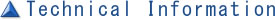##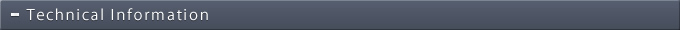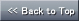### Clean / Precision Air Conditioning / Local Air Conditioning

Series Top

#### Selection method of internal circulation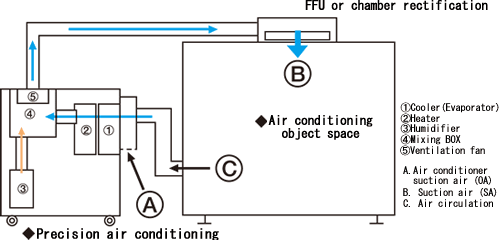Required cooling and dehumidifying formula

Air volume(m3/min)×60×enthalpy difference (k(cal/kg(DA))×air density (1.2kg (DA)/m3)÷0.86+(amount of internal heat generation (W)+endothermic due to outside air temperature (or heat dissipation))× circulation ratio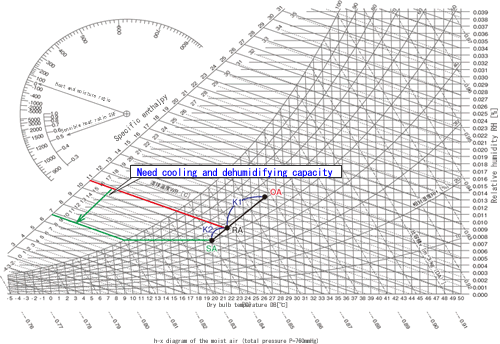Precise air conditioning heating capacity internal circulation calculation formula

Required heating capacity formula

Air volume(m3/min)×60×enthalpy difference (kcal/kg (DA))×air density (1.2kg (DA)/m3)÷0.86+(amount of internal heat generation (W)+endothermic due to outside air temperature (or heat dissipation))× circulation ratio

In case of heating, the water vapour content in the dry air does not change. Only sensible change is the heat change and this can be substituted by the below mentioned formula.

Air volume(m3/min)×60×(pre-set temperature - suction temperature)×(air density × specific heat)(0.29kg (DA)/m3)÷0.86+(amount of internal heat generation (W)+endothermic due to outside air temperature (or heat dissipation))× circulation ratio

Precise air conditioning humidification capacity internal circulation calculation formula

Required humidification capacity formula

Air volume(m3/min)×60×absolute humidity difference (kg/kg (DA))× air density (1.2kg (DA)/m3min)+ internal moisture (or humidity release)×circulating ratio

Precision air volume air conditioning requirements

Air volume standard

Air volume required is set by the size of the object space and air charge rate (times/hour) setting.

Air exchange rate (times/hour) is set by the below mentioned standard with desired precision.

*Attention! The above mentioned standard, in fact, resulting in errors due to fluctuations of both material and the outside temperature. In addition, depending on the air flow, the entire balance will change.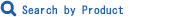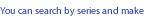Defined Search